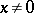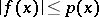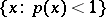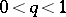A finite non-negative function $p$ on a vector space $E$ (over the field of real or complex numbers) satisfying the following conditions: \begin{equation} p(\lambda x) = |\lambda|p(x),\quad p(x+y)\leq p(x) + p(y) \end{equation} for all $x,y\in E$ and all scalars $\lambda$. An example of a semi-norm is a norm; the difference is that a semi-norm may havewith. If a semi-normis defined on a vector space and ifis a linear functional on a subspace obeying the condition, then this functional can be extended to the entire space so that the extension satisfies the same condition (the Hahn–Banach theorem). In mathematical analysis one frequently encounters separable topological vector spaces (cf. Topological vector space) in which an-neighbourhood basis exists whose elements are convex sets. Such spaces are said to be locally convex. An open convex-neighbourhood in a locally convex space is of the form, whereis a continuous semi-norm. Nevertheless, in mathematical analysis one also encounters topological vector spaces (including spaces with a metrizable topology) on which there exist no non-trivial semi-norms. The simplest examples of such spaces are,.